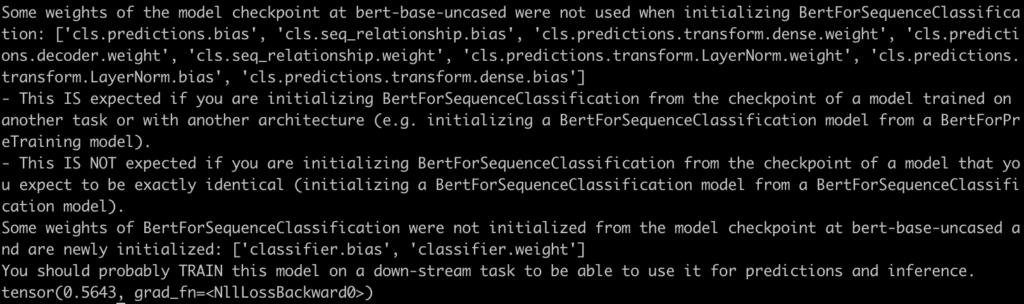# How to Fix RuntimeError: 0D or 1D target tensor expected, multi-target not supported

The RuntimeError: 0D or 1D target tensor expected, multi-target not supported error is raised when the target tensor (labels) has an incorrect shape. In PyTorch, the `CrossEntropyLoss` expects the target tensor to have a 1D shape.

To fix the RuntimeError: 0D or 1D target tensor expected, multi-target not supported,  make sure your labels tensor has the correct shape. The tensor should have a shape of (batch_size,), where batch_size is the number of samples in your input. The example assumes a single input sample, so the shape should be (1,).

``````import torch
from transformers import BertTokenizer, BertForSequenceClassification
from torch.nn import CrossEntropyLoss

model_name = "bert-base-uncased"
tokenizer = BertTokenizer.from_pretrained(model_name)
model = BertForSequenceClassification.from_pretrained(model_name)

input_text = "Your input text goes here"
tokens = tokenizer(input_text, return_tensors="pt")
input_ids = tokens["input_ids"]

# Forward pass to get the logits

labels = torch.tensor() # Assuming you have a binary classification task and a single input sample

# Compute the loss
loss_function = CrossEntropyLoss()
loss = loss_function(logits, labels)
print(loss)``````

OutputFor nn.CrossEntropyLoss, the target has to be a single number from the interval [0, #classes] instead of a one-hot encoded target vector. Your target is [1, 0], thus, PyTorch thinks you want multiple labels per input, which is unsupported.

You can replace your one-hot-encoded targets like this:

``````[1, 0] --> 0

[0, 1] --> 1``````

I hope this will fix the issue.

This site uses Akismet to reduce spam. Learn how your comment data is processed.